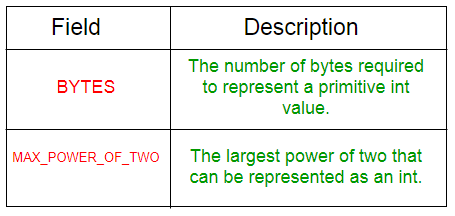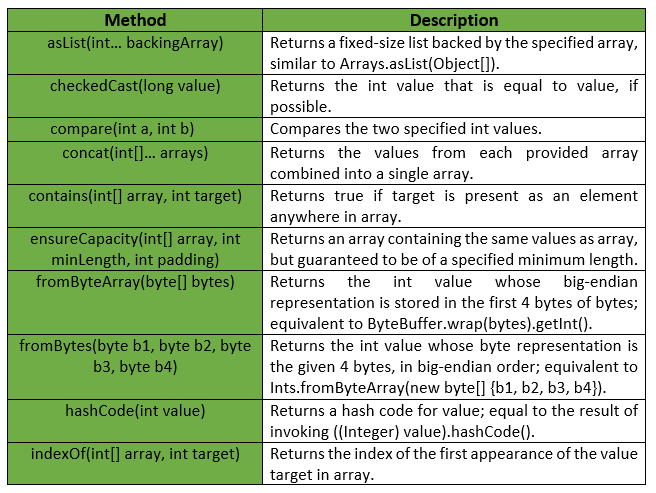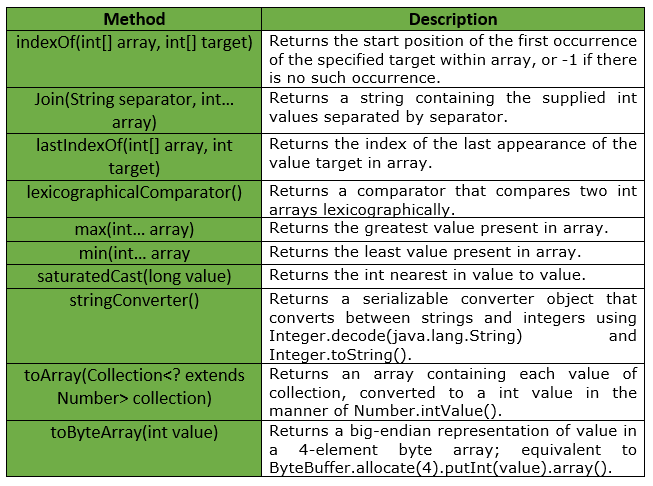# Ints Class | Guava | Java

Ints is a utility class for primitive type int. It provides Static utility methods pertaining to int primitives, that are not already found in either Integer or Arrays.

Declaration :

```@GwtCompatible(emulated=true)
public final class Ints
extends Object
```

Below table shows the Field summary for Guava Ints Class :Some of the methods provided by Ints Class are :Exceptions :

• checkedCast : IllegalArgumentException if value is greater than Integer.MAX_VALUE or less than Integer.MIN_VALUE
• min : IllegalArgumentException if array is empty.
• max : IllegalArgumentException if array is empty.
• fromByteArray : IllegalArgumentException if bytes has fewer than 4 elements.
• ensureCapacity : IllegalArgumentException if minLength or padding is negative.
• toArray : NullPointerException if collection or any of its elements is null.

Below table shows some other methods provided by Guava’s Ints Class :Below given are some examples showing the implementation of methods of Guava’s Ints Class :
Example 1 :

 `// Java code to show implementation ` `// of Guava Ints.asList() method ` ` `  `import` `com.google.common.primitives.Ints; ` `import` `java.util.*; ` ` `  `class` `GFG { ` `    ``// Driver method ` `    ``public` `static` `void` `main(String[] args) ` `    ``{ ` `        ``int` `arr[] = { ``5``, ``10``, ``15``, ``20``, ``25` `}; ` ` `  `        ``// Using Ints.asList() method which wraps ` `        ``// the primitive integer array as List of ` `        ``// integer Type ` `        ``List myList = Ints.asList(arr); ` ` `  `        ``// Displaying the elements ` `        ``System.out.println(myList); ` `    ``} ` `} `

Output :

```[5, 10, 15, 20, 25]
```

Example 2 :

 `// Java code to show implementation ` `// of Guava Ints.toArray() method ` ` `  `import` `com.google.common.primitives.Ints; ` `import` `java.util.*; ` ` `  `class` `GFG { ` `    ``// Driver method ` `    ``public` `static` `void` `main(String[] args) ` `    ``{ ` `        ``List myList = Arrays.asList(``5``, ``10``, ``15``, ``20``, ``25``); ` ` `  `        ``// Using Ints.toArray() method which ` `        ``// converts a List of Integer to an ` `        ``// array of int ` `        ``int``[] arr = Ints.toArray(myList); ` ` `  `        ``// Displaying the elements ` `        ``System.out.println(Arrays.toString(arr)); ` `    ``} ` `} `

Output :

```[5, 10, 15, 20, 25]
```

Example 3 :

 `// Java code to show implementation ` `// of Guava Ints.concat() method ` ` `  `import` `com.google.common.primitives.Ints; ` `import` `java.util.*; ` ` `  `class` `GFG { ` `    ``// Driver method ` `    ``public` `static` `void` `main(String[] args) ` `    ``{ ` `        ``int``[] arr1 = { ``5``, ``10``, ``15` `}; ` `        ``int``[] arr2 = { ``20``, ``25` `}; ` ` `  `        ``// Using Ints.concat() method which ` `        ``// combines arrays from specified ` `        ``// arrays into a single array ` `        ``int``[] arr = Ints.concat(arr1, arr2); ` ` `  `        ``// Displaying the elements ` `        ``System.out.println(Arrays.toString(arr)); ` `    ``} ` `} `

Output :

```[5, 10, 15, 20, 25]
```

Example 4 :

 `// Java code to show implementation ` `// of Guava Ints.contains() method ` ` `  `import` `com.google.common.primitives.Ints; ` ` `  `class` `GFG { ` `    ``// Driver method ` `    ``public` `static` `void` `main(String[] args) ` `    ``{ ` `        ``int``[] arr = { ``5``, ``10``, ``15``, ``20` `}; ` ` `  `        ``// Using Ints.contains() method which ` `        ``// checks if element is present in array ` `        ``// or not ` `        ``System.out.println(Ints.contains(arr, ``10``)); ` `        ``System.out.println(Ints.contains(arr, ``17``)); ` `    ``} ` `} `

output :

```true
false
```

Example 5 :

 `// Java code to show implementation ` `// of Guava Ints.min() method ` ` `  `import` `com.google.common.primitives.Ints; ` ` `  `class` `GFG { ` `    ``// Driver method ` `    ``public` `static` `void` `main(String[] args) ` `    ``{ ` `        ``int``[] arr = { ``5``, ``10``, ``15``, ``20` `}; ` ` `  `        ``// Using Ints.min() method ` `        ``System.out.println(Ints.min(arr)); ` `    ``} ` `} `

Output :

```5
```

Example 6 :

 `// Java code to show implementation ` `// of Guava Ints.max() method ` ` `  `import` `com.google.common.primitives.Ints; ` ` `  `class` `GFG { ` `    ``// Driver method ` `    ``public` `static` `void` `main(String[] args) ` `    ``{ ` `        ``int``[] arr = { ``5``, ``10``, ``15``, ``20` `}; ` ` `  `        ``// Using Ints.max() method ` `        ``System.out.println(Ints.max(arr)); ` `    ``} ` `} `

Output :

```20
```

Attention reader! Don’t stop learning now. Get hold of all the important Java and Collections concepts with the Fundamentals of Java and Java Collections Course at a student-friendly price and become industry ready.

My Personal Notes arrow_drop_upIn love with a semicolon because sometimes i miss it so badly)

If you like GeeksforGeeks and would like to contribute, you can also write an article using contribute.geeksforgeeks.org or mail your article to contribute@geeksforgeeks.org. See your article appearing on the GeeksforGeeks main page and help other Geeks.

Please Improve this article if you find anything incorrect by clicking on the "Improve Article" button below.

Article Tags :
Practice Tags :

Be the First to upvote.

Please write to us at contribute@geeksforgeeks.org to report any issue with the above content.